### PROFILE Statement

 PROFILE  parms [ / [ ALPHA= values ] [ options ] ] ;

where parms is given in the format pnam_1 pnam_2 …pnam_n, and values is the list ofvalues in (0,1).

The PROFILE statement

• writes the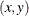coordinates of profile points for each of the listed parameters to the OUTEST= data set

• displays, or writes to the OUTEST= data set, the profile likelihood confidence limits (PL CLs) for the listed parameters for the specifiedvalues. If the approximate standard errors are available, the corresponding Wald confidence limits can be computed.

When computing the profile points or likelihood profile confidence intervals, PROC NLP assumes that a maximization of the log likelihood function is desired. Each point of the profile and each endpoint of the confidence interval is computed by solving corresponding nonlinear optimization problems.

The keyword PROFILE must be followed by the names of parameters for which the profile or the PL CLs should be computed. If the parameter name list is empty, the profiles and PL CLs for all parameters are computed. Then, optionally, thevalues follow. The list ofvalues may contain TO and BY keywords. Each element must satisfy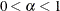. The following is an example:

   profile l11-l15 u1-u5 c /
alpha= .9 to .1 by -.1 .09 to .01 by -.01;


Duplicatevalues or values outside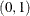are automatically eliminated from the list.

A number of additional options can be specified.

FFACTOR=specifies the factor relating the discrepancy function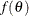to thequantile. The default value is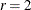.

FORCHI= F | CHI

defines the scale for the
values written to the OUTEST= data set. For FORCHI=F, thevalues are scaled to the values of the log likelihood function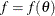; for FORCHI=CHI, thevalues are scaled so that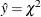. The default value is FORCHI=F.

FEASRATIO=specifies a factor of the Wald confidence limit (or an approximation of it if standard errors are not computed) defining an upper bound for the search for confidence limits. In general, the range ofvalues in the profile graph is between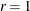andtimes the length of the corresponding Wald interval. For many examples, thequantiles corresponding to smallvalues define alevel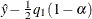, which is too far away from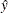to be reached by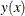forwithin the range of twice the Wald confidence limit. The search for an intersection with such alevel at a practically infinite value ofcan be computationally expensive. A smaller value forcan speed up computation time by restricting the search for confidence limits to a region closer to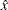. The default value of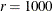practically disables the FEASRATIO= option.

OUTTABLE

specifies that the complete setof parameter estimates rather than only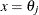for each confidence limit is written to the OUTEST= data set. This output can be helpful for further analyses on how small changes inaffect the changes in the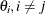.

For some applications, it may be computationally less expensive to compute the PL confidence limits for a few parameters than to compute the approximate covariance matrix of many parameters, which is the basis for the Wald confidence limits. However, the computation of the profile of the discrepancy function and the corresponding CLs in general will be much more time-consuming than that of the Wald CLs.